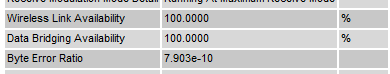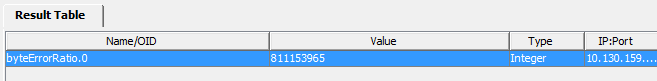# Interpreting Byte Error Ratio in the SNMP MIB

PTP 650 and PTP 700 provide a useful measure of wireless link performance in the attribute Byte Error Ratio.

Byte Error Ratio is displayed in the web-based user interface as a floating point number, like this:However, the same attribute is reported in the SNMP MIB as an IEEE754 floating point number, which is not defined in SNMP, and therefore not displayed properly in many SNMP MIB browsers. For example, here's the output using iReasoning:We need to convert the return value to a floating point number. Here's an Excel macro that does this task:

Function IEEE2Dec(dblIEEE754 As Double) As Double

Dim intSign As Integer, intExponent As Integer, dblMantissa As Double

If Int(dblIEEE754 / 2 ^ 31) = 0 Then
intSign = 1
Else
intSign = -1
dblIEEE754 = dblIEEE754 - 2 ^ 31
End If

intExponent = Int(dblIEEE754 / 2 ^ 23)

dblIEEE754 = dblIEEE754 - intExponent * 2 ^ 23

intExponent = intExponent - 127

dblMantissa = 1# + dblIEEE754 / 2 ^ 23

IEEE2Dec = intSign * 2 ^ intExponent * dblMantissa

End Function

Excel converts the returned integer value like this: IEEE2Dec(811153865) = 7.903e-10.

4 Likes